NCERT Solutions for Class 10 Mathematics Chapter 3 Pair of Linear Equations in Two Variables Ex 3.4 are part of NCERT Solutions for Class 10 Maths. Here we have given NCERT Solutions for Class 10 Mathematics Chapter 3 Pair of Linear Equations in Two Variables Ex 3.4.

 Board CBSE Textbook NCERT Class Class 10 Subject Maths Chapter Chapter 3 Chapter Name Pair of Linear Equations in Two Variables Exercise Ex 3.4 Number of Questions Solved 2 Category NCERT Solutions

### NCERT Solutions for Class 10 Maths Chapter 3 Pair of Linear Equations in Two Variables Ex 3.4

Question 1.
Solve the following pair of linear equations by the elimination method and the substitution
(i) x + y = 5 and 2x – 3y = 4
(ii) 3x + 4y = 10 and 2x – 2y = 2
(iii) 3x – 5y – 4 = 0 and 9x = 2y + 7
(iv) x/2 + 2y/3 = -1 and x – y/3 = 3
Solution:
(i) By Elimination Method:
Fquations are x + y = 5
and 2x – 3y = 4
Multiply equation (i) by 2 and subtract equation (ii) from it, we have(ii)
By Elimination method:
Equations are 3x + 4y = 10
and 2x – 2y = 2
Multiplying equation (ii) by 2 and adding to equation (i), we(iii)
By Elimination Method:(iv) By Elimination Method:
1st equation :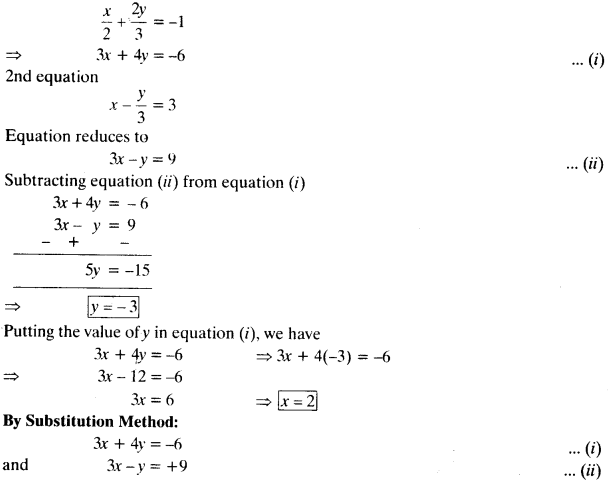Question 2.
Form the pair of linear equations in the following problems, and find their solutions (if they exist) by the elimination method:
(i) If we add 1 to the numerator and subtract 1 from the denominator, a fraction reduces to 1. It becomes – if we only add 1 to the denominator. What is the fraction₹
(ii) Five years ago, Nuri was thrice as old as Sonu. Ten years later, Nuri will be twice as old as Sonu. How old are Nuri and Sonu₹
(iii) The sum of the digits of a two-digit number is 9. Also, nine times this number is twice the number obtained by reversing the order of the digits. Find the number.
(iv) Meena went to a bank to withdraw ₹ 2000. She asked the cashier to give her ₹ 50 and ₹ 100 notes only. Meena got 25 notes in all. Find how many notes of ₹ 50 and ₹ 100 she received.
(v) A lending library has a fixed charge for the first three days and an additional charge for each day thereafter. Saritha paid ₹ 27 for a book kept for seven days, while Susy paid ₹ 21 for the book she kept for five days. Find the fixed charge and the charge for each extra day.
Solution:
(i) Let numerator be x and denominator be y.
Fraction = x/y
A.T.Q.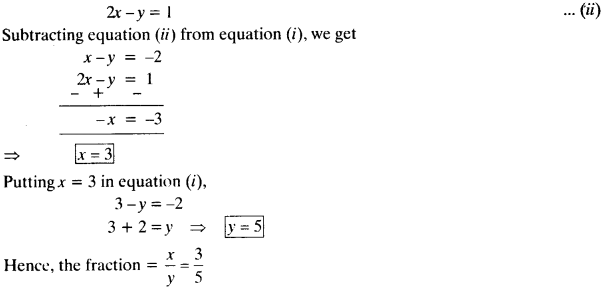(ii)
Let present age of Nuri be x years and Sonu’s present age bey years.
A.T.Q.
1st Condition :2nd Condition :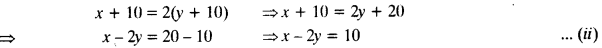Subtractomg equation (ii) from equetion (i), we get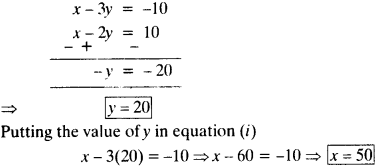Hence, present age of Nuri is 50 years and sonu’s present age is 20 years.

(iii) Let digit at unit place = x and digit at ten’s place = y.
Two digit number is lOy + x
A.T.Q.
1st Condition :
x + y = 9
2nd Condition :
9(10y + x) = 2(10k + y) ⇒ 90y + 9x = 20x + 2y
⇒ 88y – 11x = 0 ⇒ -11y + 88y = 0
⇒ -x + 8y = 0
Adding equestion (i) and (ii), we get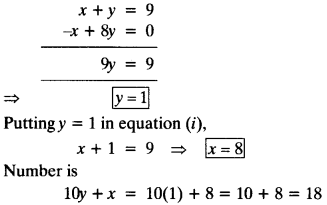(iv) Let the number of notes of ₹ 50 = x and the number of notes of ₹ 100 = y
A.T.Q
1st Condition :
50x + 100y = 2000
⇒ x + 2y = 40
2nd Condition :(v) Let, fixed charge for first 3 days be ₹ x and additional charge per day after 3 days be y.
A.T.Q.
1st Condition : as per Saritha
x + 4y = 27
2nd Condition : as per SusyPutting y = 3 in equation (i),
x + 4(3) = 27 ⇒ x + 12 = 27 ⇒ x = 15
Hence, fixed charge is ₹ 15 and charge for each extra day is ₹ 3.

We hope the NCERT Solutions for Class 10 Mathematics Chapter 3 Pair of Linear Equations in Two Variables Ex 3.4 help you. If you have any query regarding NCERT Solutions for Class 10 Mathematics Chapter 3 Pair of Linear Equations in Two Variables Ex 3.4, drop a comment below and we will get back to you at the earliest.